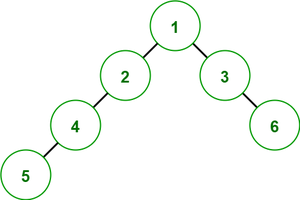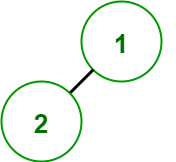Open In App

# Total time to visit all nodes of a binary tree

Given a binary tree, find the total time to visit all nodes from the root node. Visiting a child node from its parent node will cost 1 unit of time and visiting the parent from the child node will cause 0 units of time.

Examples:

Input:Output: 5
Explanation: The traversals taking 1 unit of time are 1->2, 2->4, 4->5, 1->3 and 3->6.

Input:Output: 1

Approach: The catch here is that the total time taken will be the number of edges in the binary tree. So, the answer to this problem can be found for these two cases:

Total time to visit all nodes of a binary tree if the number of nodes (N) is known: If the number of nodes in the binary tree is already known as N then the number of edges in it is N-1. So, the answer is N-1 in this case.

Time Complexity: O(1)
Auxiliary Space: O(1)

Total time to visit all nodes of a binary tree if the number of nodes is unknown: Apply dfs to get the number of edges and return it as the answer. Now follow the below steps to solve this :

1. Create a recursive function totalTime which will accept a node as the parameter and will return the number of edges below it.
2. So, get the number of edges in the left child by calling totalTime function for the left child and adding one to it.
3. Repeat the above step for the right child.
4. In the end, return the sum of edges in the left and in the right.
5. Print the answer according to the above approach.

Below is the implementation of the above approach:

## C++

 `// C++ code for the above approach`` ` `#include ``using` `namespace` `std;`` ` `// Node of the binary tree``class` `Node {``public``:``    ``int` `data;``    ``Node *left, *right;``    ``Node(``int` `d)``    ``{``        ``data = d;``        ``left = right = NULL;``    ``}``};`` ` `// Function to find the total time taken``// to visit all nodes from the root nodes``int` `totalTime(Node* root)``{`` ` `    ``int` `l = 0, r = 0;``    ``if` `(root->left) {``        ``l = totalTime(root->left) + 1;``    ``}``    ``if` `(root->right) {``        ``r = totalTime(root->right) + 1;``    ``}`` ` `    ``return` `l + r;``}`` ` `// Driver Code``int` `main()``{``    ``// Given Binary Tree``    ``Node* root = ``new` `Node(1);``    ``root->left = ``new` `Node(2);``    ``root->left->left = ``new` `Node(4);``    ``root->left->left->left = ``new` `Node(5);``    ``root->right = ``new` `Node(3);``    ``root->right->left = ``new` `Node(6);``    ``root->right->right = ``new` `Node(7);`` ` `    ``cout << totalTime(root);``    ``return` `0;``}`

## Java

 `// Java code for the above approach``import` `java.util.*;`` ` `class` `GFG{`` ` `// Node of the binary tree``static` `class` `Node {`` ` `    ``int` `data;``    ``Node left, right;``    ``Node(``int` `d)``    ``{``        ``data = d;``        ``left = right = ``null``;``    ``}``};`` ` `// Function to find the total time taken``// to visit all nodes from the root nodes``static` `int` `totalTime(Node root)``{`` ` `    ``int` `l = ``0``, r = ``0``;``    ``if` `(root.left!=``null``) {``        ``l = totalTime(root.left) + ``1``;``    ``}``    ``if` `(root.right!=``null``) {``        ``r = totalTime(root.right) + ``1``;``    ``}`` ` `    ``return` `l + r;``}`` ` `// Driver Code``public` `static` `void` `main(String[] args)``{``    ``// Given Binary Tree``    ``Node root = ``new` `Node(``1``);``    ``root.left = ``new` `Node(``2``);``    ``root.left.left = ``new` `Node(``4``);``    ``root.left.left.left = ``new` `Node(``5``);``    ``root.right = ``new` `Node(``3``);``    ``root.right.left = ``new` `Node(``6``);``    ``root.right.right = ``new` `Node(``7``);`` ` `    ``System.out.print(totalTime(root));``}``}`` ` `// This code is contributed by 29AjayKumar`

## Python3

 `# Python code for the above approach``# Node of the binary tree``class` `Node:``    ``def` `__init__(``self``, d):``        ``self``.data ``=` `d``        ``self``.left ``=` `None``        ``self``.right ``=` `None`` ` `# Function to find the total time taken``# to visit all nodes from the root nodes``def` `totalTime(root):``    ``l ``=` `0``    ``r ``=` `0``    ``if` `(root.left):``        ``l ``=` `totalTime(root.left) ``+` `1``    ``if` `(root.right):``        ``r ``=` `totalTime(root.right) ``+` `1`` ` `    ``return` `l ``+` `r`` ` `# Driver Code`` ` `# Given Binary Tree``root ``=` `Node(``1``)``root.left ``=` `Node(``2``)``root.left.left ``=` `Node(``4``)``root.left.left.left ``=` `Node(``5``)``root.right ``=` `Node(``3``)``root.right.left ``=` `Node(``6``)``root.right.right ``=` `Node(``7``)`` ` `print``(totalTime(root))`` ` `# This code is contributed by Saurabh jaiswal`

## C#

 `// C# code for the above approach``using` `System;``public` `class` `GFG{`` ` `// Node of the binary tree``class` `Node {`` ` `    ``public` `int` `data;``    ``public` `Node left, right;``    ``public` `Node(``int` `d)``    ``{``        ``data = d;``        ``left = right = ``null``;``    ``}``};`` ` `// Function to find the total time taken``// to visit all nodes from the root nodes``static` `int` `totalTime(Node root)``{`` ` `    ``int` `l = 0, r = 0;``    ``if` `(root.left!=``null``) {``        ``l = totalTime(root.left) + 1;``    ``}``    ``if` `(root.right!=``null``) {``        ``r = totalTime(root.right) + 1;``    ``}`` ` `    ``return` `l + r;``}`` ` `// Driver Code``public` `static` `void` `Main(String[] args)``{``   ` `    ``// Given Binary Tree``    ``Node root = ``new` `Node(1);``    ``root.left = ``new` `Node(2);``    ``root.left.left = ``new` `Node(4);``    ``root.left.left.left = ``new` `Node(5);``    ``root.right = ``new` `Node(3);``    ``root.right.left = ``new` `Node(6);``    ``root.right.right = ``new` `Node(7);`` ` `    ``Console.Write(totalTime(root));``}``}`` ` `// This code is contributed by 29AjayKumar`

## Javascript

 ``

Output

`6`

Time Complexity: O(V) where V is the number of nodes of the binary tree.
Auxiliary Space: O(1)